Gamasutra is part of the Informa Tech Division of Informa PLC

This site is operated by a business or businesses owned by Informa PLC and all copyright resides with them. Informa PLC's registered office is 5 Howick Place, London SW1P 1WG. Registered in England and Wales. Number 8860726.Latest Jobs
May 17, 2021Latest Blogs
May 17, 2021Press Releases
May 17, 2021
Games PressAboutGama Network
If you enjoy reading this site, you might also want to check out these UBM Tech sites:Blogs

# How to calculate the lifetime value: the review of methods

by Eugene Matveev on 09/03/15 01:12:00 pmThe following blog post, unless otherwise noted, was written by a member of Gamasutra’s community.
The thoughts and opinions expressed are those of the writer and not Gamasutra or its parent company.

The question of calculating lifetime value (also known as LTV, customer lifetime value, CLV) sooner or later arises before the developers of mobile (though not only) applications. There are many methods of LTV calculation invented, and so many men, so many minds. In this article, we decided to describe the most common methods and identify their strengths and weaknesses. These methods are primarily  suitable for describing f2p-model.

## Post Factum

This method stands out from all the following because it does not model LTV and does not predict it, and considers the actual LTV.

For this method, it is necessary to take a cohort of people who have already left the project, to see how much money the whole cohort brought and then divide that amount by the size of the cohort. It is desirable that users were registered at the same time (at the same month, but better - at the same day).

In practice, this method is poorly applicable because there is always at least one person in the cohort, who is still active, no matter how long the cohort was registered. And this is why in practice the LTV is modeled, and is not calculated as the fact. And all the following methods precisely model the future LTV, and do not assess the previous.

## Take everything and divide

The fastest, but crude method. Take the entire revenue of the application per period and divide it by the total number of users for the same period.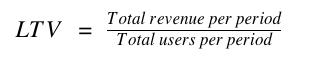Pros - for this method there is only one: it is calculated really fast, almost in a single action.

Cons caused by the obvious inaccuracy of the method, which can be induced by the following reasons, for example:

• the revenue from those users who have already become active (are in the denominator), but have not generated any revenue yet (would get in the numerator) is not taken into account;

• the calculation takes into account values of the metrics of the application from the beginning of its life; but we shouldn't forget that every application has its own lifecycle, and usually at the beginning of the lifecycle, the performance is better than some time after (see the excellent research from GameAnalytics). In this method, the stages of the lifecycle of application are looked at as one;

• in this method, it is difficult to calculate LTV for each user segment, it is necessary to know in advance the size of the segment and the amount of money brought by users from this segment.

## Lifetime in a simple way

This is the method provided by devtodev.com at the moment.

If we know how many days the average user spends in the application and how much money he brings in an average per day, we can estimate how much money he will bring for his entire life in the application. And this is our LTV. The formula of this method is as follows: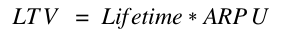Then the question arises, how to count lifetime. There are two methods, and the first one - is the calculation in a simple way (as you might have noticed from the title).

1. We define the period of inactivity, it's the time after which the user is most likely not to return to the application. It is determined either on the basis of retention values, or, which is more often, expertly. Usually it is set expertly to the value of one or two weeks.

2. Every day we look at the users who have their period of inactivity expired at this particular day.

3. For each user, we calculate the number of days from his first visit to the current date.

We count the average value for all users. This is lifetime.

So ARPU (in this case, ARPU = ARPDAU) is calculated as the daily Revenue, divided by DAU. To get LTV just multiply lifetime by ARPU.

Pros of the method:

• The simplicity of calculations. Calculate lifetime by this method is easy, and even easier to calculate ARPU. And any schoolboy can multiply one by the other.

• You may calculate LTV every day if you want to.

• LTV can be calculated for each user segment individually.

Cons are again in inaccuracy, which in this case is due to the following reasons:

• The value depends strongly on the inactivity period, which as a rule is defined expertly.

• We multiply the average value of lifetime by the average value of ARPU and get the  accumulated fallacy.

• When calculating lifetime we look at those users who already left the application. When calculating ARPU, we look at the users of the current day. It turns out that the plurality of users forming lifetime and ARPU, do not intersect: lifetime is calculated according to the data of the past days, ARPU - to the data of the current day.

• There is a strong assumption of the immutability of ARPU. We take ARPU for only one day, and on its basis we model LTV for many days ahead.

## Lifetime in a complex way, or Bottoms Up

The second name of this method comes from the Wooga article, and this will agree, a source which is worth considering. The formula for this method is the same:But lifetime here calculated a bit more complicated and it turns out to be far more accurate. Let's recall how the retention graph looks like (image is taken from here):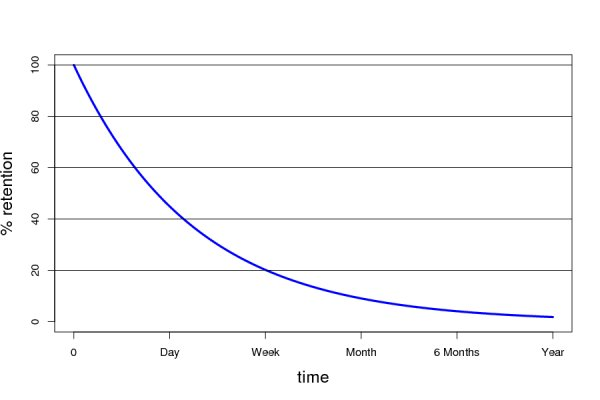In fact,  lifetime is the area under the curve of the retention graph, in other words - the integral from the retention by time.

But before we start calculating the integral, it is necessary to construct the function. This is how it's done:

1. As a rule, you have the values of retention for a few days (for example, 1 day, 7 days, 28 days). If there are any other days, and even better - longer periods of time - that's fine, it will make calculations even more accurate.

2. On the basis of the known values (for example, 1, 7 and 28 days), we need to construct a retention curve. We shall search the equation of a curve of the form: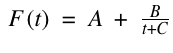where t - is the number of days from the first visit, F(t) - the future retention equation, and A, B and C - the model coefficients.

1. Substitute the known values of retention, no matter how many there are, into the equation, and get a system of equations for the coefficients A, B and C.

2. Count the sum of squared differences of the deviations between the actual and modeled values of F(t).

3. Find the values of A, B and C, which minimizes the cumulative deviation. It is perfectly easy to perform, for example, with the tool "Solver" in MS Excel.

4. Substitute the obtained values of A, B, C in the equation and obtain the function, which can be used to evaluate the retention of as many days as needed.

That's not all of it, but we're getting close. Further, you may still choose a complex or a simple method.

The complex method is to find the integral of the retention function: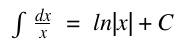The simple method consists in, even approximately, dividing the retention curve into segments depending on the lifetime value. For example: users who left after a day, users who spent in the app from 2 to 7 days, from 8 to 30 days, from 1 to 3 months, more than 3 months. The more segments the better. For each segment calculate by the retention table the percentage of users (segment weight) related to it, and then calculate the average lifetime of all segments.

But whatever method you choose, you will encounter the question, to what point LTV should be calculated (in case of the integral it will be the right edge of the field of integration, in case of the sum - the number of days in the last segment). Here again, there are two methods of solution: simple and complex.

A simple method consists in the fact that the right edge is given expertly. Typically, this occurs as follows:

• let’s take half a year!

• why?

• why not?

• ok, let’s take half a year.

The complex method is to use the discount and finding the discount rate of WACC (admit it, you did not expect to see financial math in this material?). The fact is that a thousand of dollars now and a thousand of dollars tomorrow - are the different amounts of money. The "tomorrow thousand of dollars" today will be equal to nine hundred dollars or so, depending on the choice of the discount rate.

The formula is: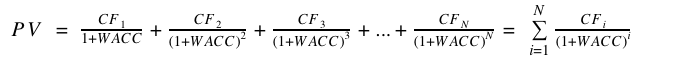Here PV (present value) - is the present value of the future money,

CFi - money, yhat you’ll get in i periods of time,

WACC (weighted average cost of capital) - the discount rate.

How to find it? Usually WACC is made to be the actual average return on equity for the company. It is also possible to equate it to the desired return on equity or to the return on equity of the alternative projects. If you do not understand this paragraph, ask your financiers, they are sure to know your company's WACC.

So, knowing the WACC, you will be able to discount the future time flows, and hence to select even infinity as the right edge of the integration. The fact is that the addition of your WACC makes the infinite descending sequence from your sum (or from your integral) that can be found as the sum.

We assume that we calculated lifetime. Now we calculate ARPU (Revenue/DAU), multiply ARPU by lifetime and get LTV.

Pros of the method:

• Accuracy. Lifetime is calculated very accurate, the fallacy in it is minimal.

• A side effect of the calculation by this method is the bonus of retention forecast for as many days as you want.

• The ability to calculate LTV for each segment separately.

Cons of the method:

• It is complicated to calculate (though an experienced analyst will calculate your LTV in five minutes in the means of the presence of all the data).

• Again, the assumption of the immutability of ARPU over time. You can play it safe and take in the calculation not ARPU for one day,  but the daily average of ARPU for the lifetime, it will increase the accuracy.

## Cumulative ARPU, or Top Down

The second method name is again taken from the Wooga article, which gives +10 to the trust of this method. The picture is taken from the same article: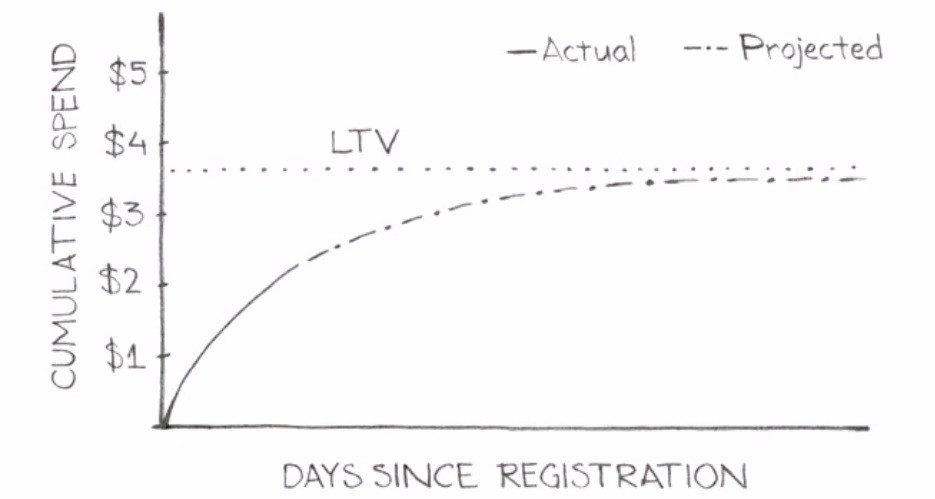Let us explain. Let's assume a group of new players came to your project, and you started to track it. You are measuring how much money is brought to you by an average player from this group in 7, 14, 28 days and so on. That is, in fact, you come from the usual
ARPU to the Cumulative ARPU for N days.

So, knowing Cumulative ARPU for 7, 14, 28, etc. days, again we will be able to build the mathematical model of the curve that will forecast the value of Cumulative ARPU for any number of days. We shall search the equation of a curve of the form: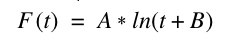where t - is the number of days from the first visit of the user, F(t) - is the future equation, A and B - are the model coefficients.

Again we calculate the sum of squared deviations and minimize it by choosing the optimal values of A and B coefficients.

If you have more values of Cumulative ARPU (say, for 60 and 90 days), you may add   extra summands of the form C*t or D/t in the equation, this shall increase the accuracy. Well, in general - there is no single equation, that gives guaranteed minimum of deviation. Experiment with the forms of the equation!

Through several iterations, you will get the equation that will suit you. Now, by substituting the value you want for t in this equation, you get Cumulative ARPU(t), that is LTV essentially.

How to choose the value for t to calculate LTV?

1. Firstly, you may take lifetime.

2. Secondly, you may again set t expertly.

3. Thirdly, you may go back to discounting and add to the resulting equation the denominator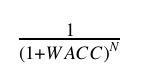In this case, sooner or later, the graph will take the shape of asymptotic value (as at the picture above - about \$ 3.7, above which the LTV will not go). This is the value you should take.

devtodev.com now develops the new section of LTV forecasting based on this method. Watch for updates!

So, we reviewed five methods of calculating LTV, as you can see, in order from the least to the most accurate. Choose the method that you like, count your LTV and make the right decisions. And now the main rule of LTV: divide users into segments, and count LTV for each segment separately. This will give you more accuracy, and more reasons to make the right decision for your product.